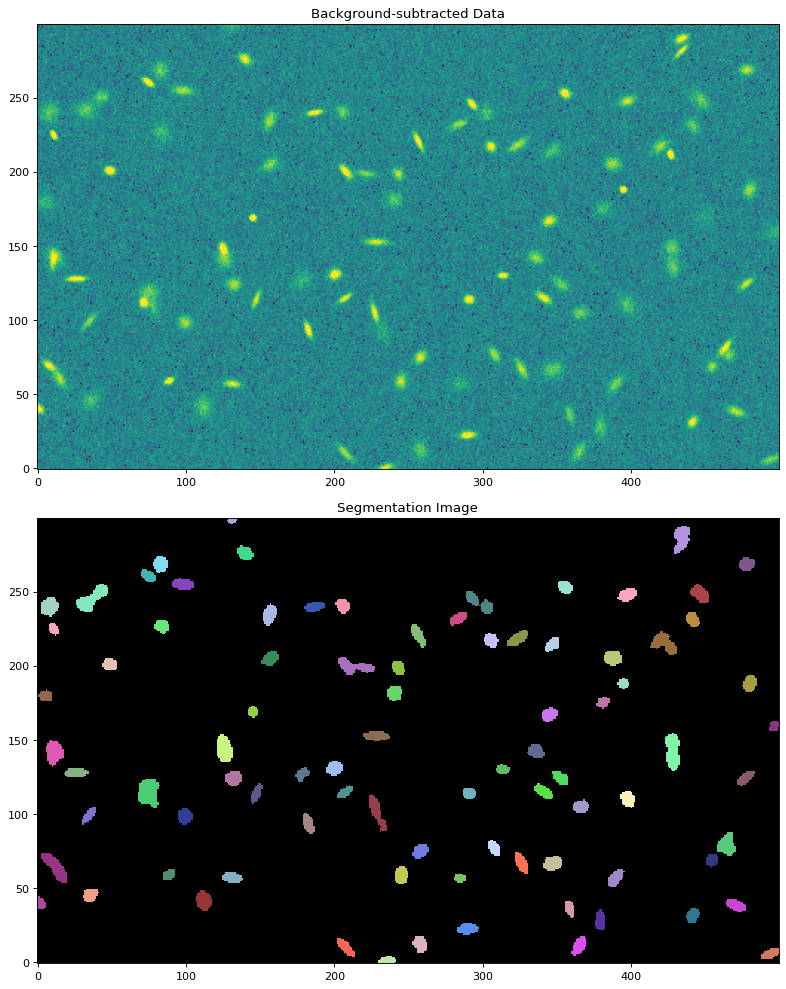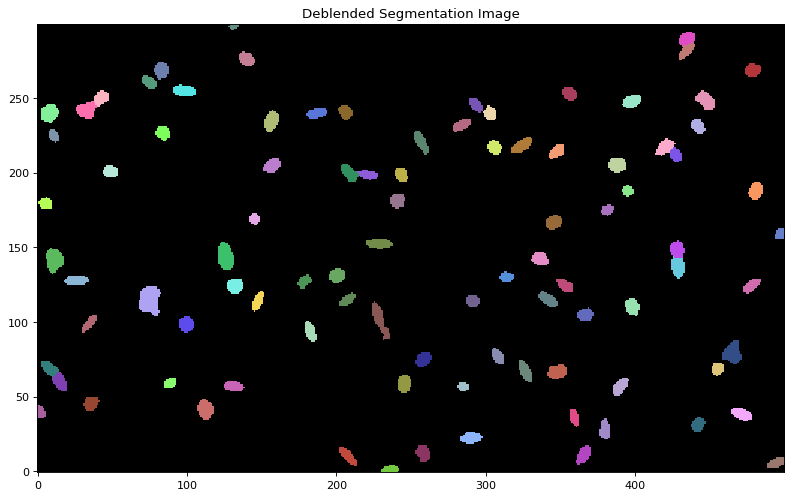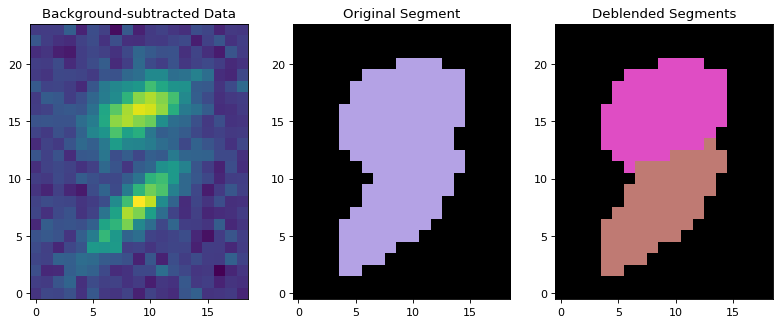# Image Segmentation (`photutils.segmentation`)¶

## Introduction¶

Photutils includes a general-use function to detect sources (both point-like and extended) in an image using a process called image segmentation. After detecting sources using image segmentation, we can then measure their photometry, centroids, and morphological properties by using additional tools in Photutils.

## Source Extraction Using Image Segmentation¶

Photutils provides tools to detect astronomical sources using image segmentation, which is a process of assigning a label to every pixel in an image such that pixels with the same label are part of the same source. Detected sources must have a minimum number of connected pixels that are each greater than a specified threshold value in an image. The threshold level is usually defined at some multiple of the background noise (sigma) above the background. The image can also be filtered before thresholding to smooth the noise and maximize the detectability of objects with a shape similar to the filter kernel.

Let’s start by detecting sources in a synthetic image provided by the photutils.datasets module:

```>>> from photutils.datasets import make_100gaussians_image
>>> data = make_100gaussians_image()
```

The source segmentation/extraction is performed using the `detect_sources()` function. We will use a convenience function called `detect_threshold()` to produce a 2D detection threshold image using simple sigma-clipped statistics to estimate the background level and RMS.

The threshold level is calculated using the `nsigma` input as the number of standard deviations (per pixel) above the background. Here we generate a simple threshold at 2 sigma (per pixel) above the background:

```>>> from photutils.segmentation import detect_threshold
>>> threshold = detect_threshold(data, nsigma=2.)
```

For more sophisticated analyses, one should generate a 2D background and background-only error image (e.g., from your data reduction or by using `Background2D`). In that case, a 2-sigma threshold image is simply:

```>>> threshold = bkg + (2.0 * bkg_rms)
```

Note that if the threshold includes the background level (as above), then the image input into `detect_sources()` should not be background subtracted. In other words, the input threshold value(s) are compared directly to the input image. Because the threshold returned by `detect_threshold()` includes the background, we do not subtract the background from the data here.

Let’s find sources that have 5 connected pixels that are each greater than the corresponding pixel-wise `threshold` level defined above (i.e., 2 sigma per pixel above the background noise). Note that by default “connected pixels” means “8-connected” pixels, where pixels touch along their edges or corners. One can also use “4-connected” pixels that touch only along their edges by setting `connectivity=4` in `detect_sources()`.

We will also input a 2D circular Gaussian kernel with a FWHM of 3 pixels to smooth the image prior to thresholding:

```>>> from astropy.convolution import Gaussian2DKernel
>>> from astropy.stats import gaussian_fwhm_to_sigma
>>> from photutils.segmentation import detect_sources
>>> sigma = 3.0 * gaussian_fwhm_to_sigma  # FWHM = 3.
>>> kernel = Gaussian2DKernel(sigma, x_size=3, y_size=3)
>>> kernel.normalize()
>>> segm = detect_sources(data, threshold, npixels=5, filter_kernel=kernel)
```

The result is a `SegmentationImage` object with the same shape as the data, where detected sources are labeled by different positive integer values. A value of zero is always reserved for the background. Let’s plot both the image and the segmentation image showing the detected sources:

```>>> import numpy as np
>>> import matplotlib.pyplot as plt
>>> from astropy.visualization import SqrtStretch
>>> from astropy.visualization.mpl_normalize import ImageNormalize
>>> norm = ImageNormalize(stretch=SqrtStretch())
>>> fig, (ax1, ax2) = plt.subplots(2, 1, figsize=(10, 12.5))
>>> ax1.imshow(data, origin='lower', cmap='Greys_r', norm=norm)
>>> ax1.set_title('Data')
>>> cmap = segm.make_cmap(seed=123)
>>> ax2.imshow(segm, origin='lower', cmap=cmap, interpolation='nearest')
>>> ax2.set_title('Segmentation Image')
```When the segmentation image is generated using image thresholding (e.g., using `detect_sources()`), the source segments represent the isophotal footprints of each source.

## Source Deblending¶

In the example above, overlapping sources are detected as single sources. Separating those sources requires a deblending procedure, such as a multi-thresholding technique used by SourceExtractor. Photutils provides a `deblend_sources()` function that deblends sources uses a combination of multi-thresholding and watershed segmentation. Note that in order to deblend sources, they must be separated enough such that there is a saddle between them.

The amount of deblending can be controlled with the two `deblend_sources()` keywords `nlevels` and `contrast`. `nlevels` is the number of multi-thresholding levels to use. `contrast` is the fraction of the total source flux that a local peak must have to be considered as a separate object.

Here’s a simple example of source deblending:

```>>> from photutils.segmentation import deblend_sources
>>> segm_deblend = deblend_sources(data, segm, npixels=5,
...                                filter_kernel=kernel, nlevels=32,
...                                contrast=0.001)
```

where `segm` is the `SegmentationImage` that was generated by `detect_sources()`. Note that the `npixels` and `filter_kernel` input values should match those used in `detect_sources()` to generate `segm`. The result is a new `SegmentationImage` object containing the deblended segmentation image:Let’s plot one of the deblended sources:## Modifying a Segmentation Image¶

The `SegmentationImage` object provides several methods that can be used to visualize or modify itself (e.g., combining labels, removing labels, removing border segments) prior to measuring source photometry and other source properties, including:

## Centroids, Photometry, and Morphological Properties¶

The `SourceCatalog` class is the primary tool for measuring the centroids, photometry, and morphological properties of sources defined in a segmentation image. When the segmentation image is generated using image thresholding (e.g., using `detect_sources()`), the source segments represent the isophotal footprint of each source and the resulting photometry is effectively isophotal photometry.

The source properties can be accessed using `SourceCatalog` attributes or output to an Astropy `QTable` using the `to_table()` method. Please see `SourceCatalog` for the the many properties that can be calculated for each source. More properties are likely to be added in the future.

Let’s detect sources and measure their properties in a synthetic image. For this example, we will use the `Background2D` class to produce a background and background noise image. We define a 2D detection threshold image using the background and background RMS images. We set the threshold at 2 sigma (per pixel) above the background:

```>>> from astropy.convolution import Gaussian2DKernel
>>> from photutils.datasets import make_100gaussians_image
>>> from photutils.background import Background2D, MedianBackground
>>> from photutils.segmentation import detect_threshold, detect_sources
>>> data = make_100gaussians_image()
>>> bkg_estimator = MedianBackground()
>>> bkg = Background2D(data, (50, 50), filter_size=(3, 3),
...                    bkg_estimator=bkg_estimator)
>>> data -= bkg.background  # subtract the background
>>> threshold = 2. * bkg.background_rms  # above the background
```

Now we find sources that have 5 connected pixels that are each greater than the corresponding threshold image defined above. Because the threshold includes the background, we do not subtract the background from the data here. We also input a 2D circular Gaussian kernel with a FWHM of 3 pixels to filter the image prior to thresholding:

```>>> from astropy.stats import gaussian_fwhm_to_sigma
>>> sigma = 3.0 * gaussian_fwhm_to_sigma  # FWHM = 3.
>>> kernel = Gaussian2DKernel(sigma, x_size=3, y_size=3)
>>> kernel.normalize()
>>> npixels = 5
>>> segm = detect_sources(data, threshold, npixels=npixels,
...                       filter_kernel=kernel)
>>> segm_deblend = deblend_sources(data, segm, npixels=npixels,
...                                filter_kernel=kernel, nlevels=32,
...                                contrast=0.001)
```

As described earlier, the result is a `SegmentationImage` where sources are labeled by different positive integer values.

Now let’s measure the properties of the detected sources defined in the segmentation image using the simplest call to `SourceCatalog`. The output `QTable` of source properties is generated by the `SourceCatalog` `to_table()` method. Each row in the table represents a source. The columns represent the calculated source properties. Note that the only a subset of the source properties are shown below. Please see `SourceCatalog` for the list of the many properties that are calculated for each source:

```>>> from photutils.segmentation import SourceCatalog
>>> cat = SourceCatalog(data, segm_deblend)
>>> tbl = cat.to_table()
>>> tbl['xcentroid'].info.format = '.2f'  # optional format
>>> tbl['ycentroid'].info.format = '.2f'
>>> tbl['kron_flux'].info.format = '.2f'
>>> print(tbl)
label xcentroid ycentroid ... segment_fluxerr kron_flux kron_fluxerr
...
----- --------- --------- ... --------------- --------- ------------
1    235.16      1.10 ...             nan    511.47          nan
2    494.16      5.82 ...             nan    541.53          nan
3    207.29     10.04 ...             nan    692.64          nan
4    364.73     11.12 ...             nan    695.36          nan
5    258.36     11.79 ...             nan    667.71          nan
...       ...       ... ...             ...       ...          ...
92    427.01    147.46 ...             nan    888.12          nan
93    426.63    211.10 ...             nan    893.63          nan
94    419.74    216.64 ...             nan    866.92          nan
95    433.95    280.71 ...             nan    631.41          nan
96    434.09    288.93 ...             nan    930.15          nan
Length = 96 rows
```

The error columns are NaN because we did not input an error array (see the Photometric Errors section below).

Let’s now plot the calculated elliptical Kron apertures (based on the shapes for of each source) on the data:

```>>> import numpy as np
>>> import matplotlib.pyplot as plt
>>> from astropy.visualization import simple_norm
>>> norm = simple_norm(data, 'sqrt')
>>> fig, (ax1, ax2) = plt.subplots(2, 1, figsize=(10, 12.5))
>>> ax1.imshow(data, origin='lower', cmap='Greys_r', norm=norm)
>>> ax1.set_title('Data')
>>> cmap = segm_deblend.make_cmap(seed=123)
>>> ax2.imshow(segm_deblend, origin='lower', cmap=cmap,
...            interpolation='nearest')
>>> ax2.set_title('Segmentation Image')
>>> for aperture in cat.kron_aperture:
...     aperture.plot(axes=ax1, color='white', lw=1.5)
...     aperture.plot(axes=ax2, color='white', lw=1.5)
```We can also create a `SourceCatalog` object containing only a specific subset of sources, defined by their label numbers in the segmentation image:

```>>> cat = SourceCatalog(data, segm_deblend)
>>> labels = [1, 5, 20, 50, 75, 80]
>>> cat_subset = cat.get_labels(labels)
>>> tbl2 = cat_subset.to_table()
>>> tbl2['xcentroid'].info.format = '.2f'  # optional format
>>> tbl2['ycentroid'].info.format = '.2f'
>>> tbl2['kron_flux'].info.format = '.2f'
>>> print(tbl2)
label xcentroid ycentroid ... segment_fluxerr kron_flux kron_fluxerr
...
----- --------- --------- ... --------------- --------- ------------
1    235.16      1.10 ...             nan    511.47          nan
5    258.36     11.79 ...             nan    667.71          nan
20    347.02     66.92 ...             nan    815.06          nan
50    145.06    168.54 ...             nan    715.61          nan
75    301.87    239.26 ...             nan    512.10          nan
80     43.25    250.03 ...             nan    662.00          nan
```

By default, the `to_table()` includes only a small subset of source properties. The output table properties can be specified (or excluded) in the `QTable` via the `columns` or `exclude_columns` keywords:

```>>> cat = SourceCatalog(data, segm_deblend)
>>> labels = [1, 5, 20, 50, 75, 80]
>>> cat_subset = cat.get_labels(labels)
>>> columns = ['label', 'xcentroid', 'ycentroid', 'area', 'segment_flux']
>>> tbl3 = cat_subset.to_table(columns=columns)
>>> tbl3['xcentroid'].info.format = '.4f'  # optional format
>>> tbl3['ycentroid'].info.format = '.4f'
>>> tbl3['segment_flux'].info.format = '.4f'
>>> print(tbl3)
label xcentroid ycentroid area segment_flux
pix2
----- --------- --------- ---- ------------
1  235.1599    1.1019 38.0     421.5506
5  258.3583   11.7944 59.0     384.3829
20  347.0213   66.9189 73.0     479.6836
50  145.0619  168.5415 33.0     714.7382
75  301.8673  239.2567 36.0     206.0899
80   43.2456  250.0334 56.0     342.9081
```

A `WCS` transformation can also be input to `SourceCatalog` via the `wcs` keyword, in which case the sky coordinates of the source centroids can be calculated.

### Background Properties¶

Like with `aperture_photometry()`, the `data` array that is input to `SourceCatalog` should be background subtracted. If you input the background image that was subtracted from the data into the `background` keyword of `SourceCatalog`, the background properties for each source will also be calculated:

```>>> cat = SourceCatalog(data, segm_deblend, background=bkg.background)
>>> labels = [1, 5, 20, 50, 75, 80]
>>> cat_subset = cat.get_labels(labels)
>>> columns = ['label', 'background_centroid', 'background_mean',
...            'background_sum']
>>> tbl4 = cat_subset.to_table(columns=columns)
>>> tbl4['background_centroid'].info.format = '{:.10f}'  # optional format
>>> tbl4['background_mean'].info.format = '{:.10f}'
>>> tbl4['background_sum'].info.format = '{:.10f}'
>>> print(tbl4)
label background_centroid background_mean background_sum
----- ------------------- --------------- --------------
1        5.2045930090    5.2021426705 197.6814214808
5        5.2161445822    5.2102818673 307.4066301727
20        5.2363604148    5.2780021156 385.2941544392
50        5.1752766952    5.1884834993 171.2199554780
75        5.1101563277    5.1409912451 185.0756848238
80        5.1934988972    5.2106591322 291.7969114012
```

### Photometric Errors¶

`SourceCatalog` requires inputting a total error array, i.e., the background-only error plus Poisson noise due to individual sources. The `calc_total_error()` function can be used to calculate the total error array from a background-only error array and an effective gain.

The `effective_gain`, which is the ratio of counts (electrons or photons) to the units of the data, is used to include the Poisson noise from the sources. `effective_gain` can either be a scalar value or a 2D image with the same shape as the `data`. A 2D effective gain image is useful for mosaic images that have variable depths (i.e., exposure times) across the field. For example, one should use an exposure-time map as the `effective_gain` for a variable depth mosaic image in count-rate units.

Let’s assume our synthetic data is in units of electrons per second. In that case, the `effective_gain` should be the exposure time (here we set it to 500 seconds). Here we use `calc_total_error()` to calculate the total error and input it into the `SourceCatalog` class. When a total `error` is input, the `segment_fluxerr` property is calculated. `segment_flux` and `segment_fluxerr` are the instrumental flux and propagated flux error within the source segments:

```>>> from photutils.utils import calc_total_error
>>> effective_gain = 500.
>>> error = calc_total_error(data, bkg.background_rms, effective_gain)
>>> cat = SourceCatalog(data, segm_deblend, error=error)
>>> labels = [1, 5, 20, 50, 75, 80]
>>> cat_subset = cat.get_labels(labels)  # select a subset of objects
>>> columns = ['label', 'xcentroid', 'ycentroid', 'segment_flux',
...            'segment_fluxerr']
>>> tbl5 = cat_subset.to_table(columns=columns)
>>> tbl5['xcentroid'].info.format = '{:.4f}'  # optional format
>>> tbl5['ycentroid'].info.format = '{:.4f}'
>>> tbl5['segment_flux'].info.format = '{:.4f}'
>>> tbl5['segment_fluxerr'].info.format = '{:.4f}'
>>> for col in tbl5.colnames:
...     tbl5[col].info.format = '%.8g'  # for consistent table output
>>> print(tbl5)
label xcentroid ycentroid segment_flux segment_fluxerr
----- --------- --------- ------------ ---------------
1 235.15995 1.1019364    421.55062       13.119038
5 258.35831 11.794386    384.38289       16.438836
20 347.02128 66.918889    479.68361       18.646413
50 145.06195 168.54152    714.73824       11.890069
75 301.86728 239.25667     206.0899       12.065216
80 43.245594 250.03337    342.90807       15.908175
```

### Pixel Masking¶

Pixels can be completely ignored/excluded (e.g., bad pixels) when measuring the source properties by providing a boolean mask image via the `mask` keyword (`True` pixel values are masked) to the `SourceCatalog` class.

### Filtering¶

SourceExtractor’s centroid and morphological parameters are calculated from a filtered “detection” image. The usual downside of the filtering is the sources will be made more circular than they actually are (assuming a circular kernel is used, which is common). If you wish to reproduce SourceExtractor results, then use the `SourceCatalog` `kernel` keyword to filter the `data` prior to centroid and morphological measurements. The kernel should be the same one used with `detect_sources()` that defined the segmentation image. If `kernel` is `None`, then the centroid and morphological measurements will be performed on the unfiltered `data`. Note that photometry is always performed on the unfiltered `data`.

## Reference/API¶

This subpackage contains tools for detecting sources using image segmentation and measuring their centroids, photometry, and morphological properties.

### Functions¶

 `deblend_sources`(data, segment_img, npixels) Deblend overlapping sources labeled in a segmentation image. `detect_sources`(data, threshold, npixels[, …]) Detect sources above a specified threshold value in an image and return a `SegmentationImage` object. `detect_threshold`(data, nsigma[, background, …]) Calculate a pixel-wise threshold image that can be used to detect sources. `make_source_mask`(data, nsigma, npixels[, …]) Make a source mask using source segmentation and binary dilation. `source_properties`(data, segment_img[, …]) Deprecated since version 1.1.

### Classes¶

 `LegacySourceCatalog`(properties_list[, wcs]) Deprecated since version 1.1. `Segment`(segment_data, label, slices, bbox, area) Class for a single labeled region (segment) within a segmentation image. Class for a segmentation image. `SourceCatalog`(data, segment_img, *[, error, …]) Class to create a catalog of photometry and morphological properties for sources defined by a segmentation image. `SourceProperties`(data, segment_img, label[, …]) Deprecated since version 1.1.